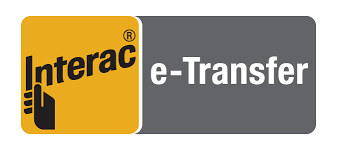# The Odds of Making a Two Pair Hand in Poker

Font Size
A A A

The odds of flopping Two Pair with an unpaired starting hand is 2.02 or 1 in 49.5

Definition of Two Pair

Two Pair – Two sets of cards of identical rank. (i.e. two one pair type hands made together).

Example – AAKKQ

Two Pair in Hold’em can be where we hold a pair in our starting hand, and there is a pair on the board, or we can pair each of our starting cards with two on the board.

## Odds of Making Two Pair on the Flop

Let’s assume we have any one of the 1,248 unpaired starting hands.

What are the odds of flopping exactly 2 Pair?

Odd of flopping exactly 2 Pair with an unpaired starting hand = 2.02%

This means that we’ll flop Two Pair roughly once in every fifty flops we see with an unpaired starting hand. This does not change depending on the starting hand, i.e. AKo flops Two Pair just as frequently as T9s.

What about the odds of flopping Two Pair or stronger?

Odds of flopping 2 Pair or better with an unpaired starting hand = 4.1%

So, although it’s relatively unlikely we’ll flop exactly 2 Pair, we’ll actually flop Two Pair or better roughly once in every 25 flops we see.

Unlike flopping Two Pair, the Two Pair plus odds, do change based on the type of hand.

AKo to flop 2 Pair plus = 3.8%

9Ts to flop 2 Pair plus = 5.6%

Q3s to flop 2 Pair plus – 4.31%

We can see that suited connected type holdings are typically in the best position to make Two Pair plus with a high frequency.

## Odds of Making Two Pair on the Later Streets

Let’s imagine that we have flopped a pair and want to know the odds of making Two Pair by the river.

By the flop, there are 47 unknown cards left in the deck, and we have 3 outs to make Two Pair. We also have 2 outs to make Trips, which are very relevant, and we’ll account for those too.

From Flop to Turn

One pair with the board…

3 out of 47 cards make us Two Pair. 3/47 = 0.0638 or roughly 6.4%

5 out of 47 cards make us Two Pair plus. 5/47 = 0.1064 or roughly 10.6%

From Turn to River

3 out of 46 cards make us Two Pair. 3/46 = 0.0652 or roughly 6.5%

5 out of 46 cards make us Two Pair plus. 5/46 = 0.1087 or roughly 10.9%

From Flop to River

To calculate this, we first enumerate the possibility of not hitting at all by the river and then subtract from 100%.

One pair with the board….

Probability of not hitting Two Pair on the turn = 44/47

Probability of not hitting Two Pair on the river = 43/46

Probability of not hitting Two Pair or Trips on the turn = 42/47

Probability of not hitting Two Pair or Trips on the river = 41/46

Probability of not hitting Two Pair on the turn or river = (44/47 * 43/46) = 0.8751 or roughly 88%

Probability of not hitting Two Pair or Trips on the turn or river= (42/47) * (41/46) = 0.7965 or roughly 79.7%

Therefore -

Probability of hitting Two Pair from flop to river = roughly 12%

Probability of hitting Two Pair or Trips from flop to river = roughly 20.3%

## Implied Odds Analysis of Two Pair

Making Two Pair often means we’ll benefit from a small amount of implied odds. We’ll usually have the best hand and can frequently either call down on the later streets or make additional value bets ourselves.

These hands are not invulnerable, however, and don’t carry the same implied odds that hands such as Straights and Flushes do. Two Pair can never be the stone-cold nuts since there is always the possibility that our opponent has a set.

Naturally, some Two Pair type hands are better than others. When making estimates regarding our implied odds we should usually think about the following -

- Do we have Top Two Pair? Top and middle? Two middle pairs? Bottom two?

Top two carries the best implied odds since our opponent might have made a dominated Two Pair.

- Is the board texture dry, or does it allow for completed draws?

Our implied odds suffer significantly on board textures where Straights and Flushes are possible. On extremely connected textures such as four-to-a-flush and four-to-a-straight textures, our Two Pair hands actually start to suffer from reverse implied odds.

Two Pair is generally a strong hand in Hold’em and is frequently strong enough to play for stacks, assuming 100bb effective.

However, we should not blindly stack off with such holdings. On textures where possible draws have already completed, we will want to exercise caution. We should also look carefully at the absolute strength of our Two Pair. Top Two Pair is definitely more valuable than bottom Two Pair.

 Method (Two Pair) Probability (%) Flopping Two Pair with an unpaired starting hand. 2.02 Flopping Two Pair or better with AKo 3.8 Flopping Two Pair or better with T9s 5.6 Flopping Two Pair or better with Q3s 4.31 Hitting Two Pair from flop to turn with a Pair 6.4 Hitting Two Pair or better from flop to turn with a Pair 10.6 Hitting Two Pair from turn to river with a Pair 6.5 Hitting Two Pair or better from turn to river with a Pair 10.9 Hitting Two Pair from flop to river with a Pair 12 Hitting Two Pair or better from flop ti river with a Pair 20.3
Did you find this interesting?

Thank you for helping us improve

Awards